# An example of the application of the Itô Rule

How does the Itô rule get applied ?

Recall the rule is: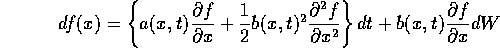Consider the SDE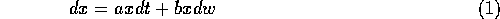and we want to introduce the change of variables,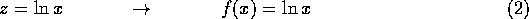So the application of the Itô rule gives,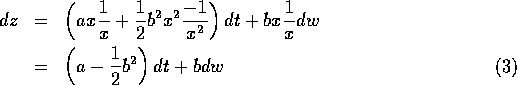For the Stratnovich case we have two equations we can consider.

First, the equation that is equivalent to the Itô system,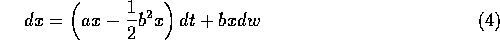The ordinary chain rule applies to this equation, so the change of variables gives us,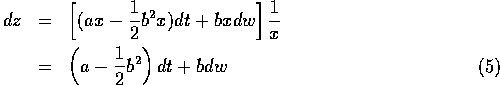which is the same as (3).

The second Stratnovich equation that we could consider, is the one that has the same form as (1). Applying the ordinary chain rule to it gives,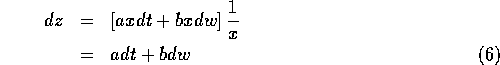which is a very different system.Next: Numerical integration of Itô SDEs Up: Equivalence of Itô and Stratonovich Calculus Previous: Equivalence of Itô and Stratonovich Calculus

Skip Carter
Thu Dec 28 10:08:17 PST 1995